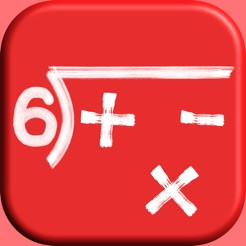## Screenshots

•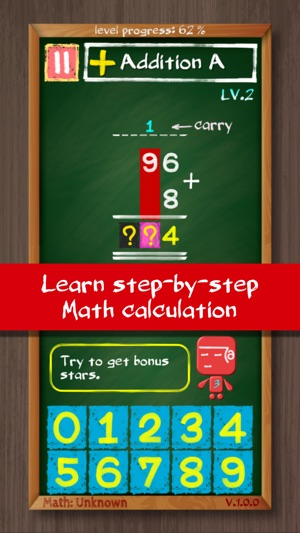•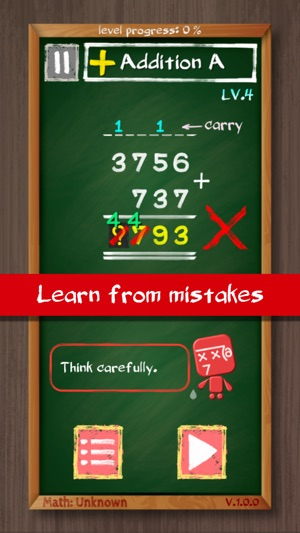•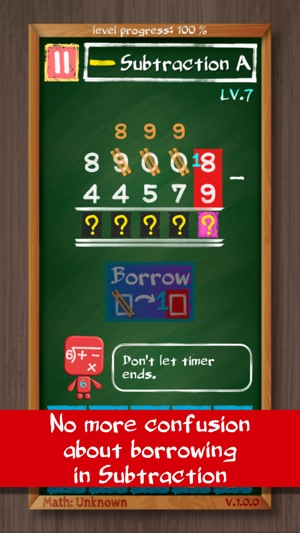•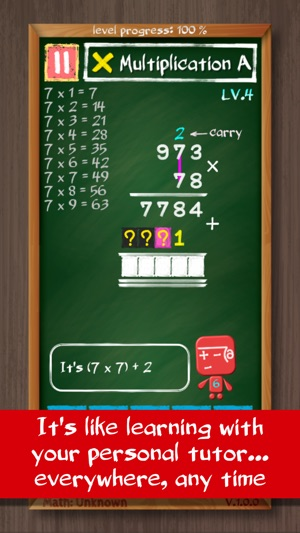•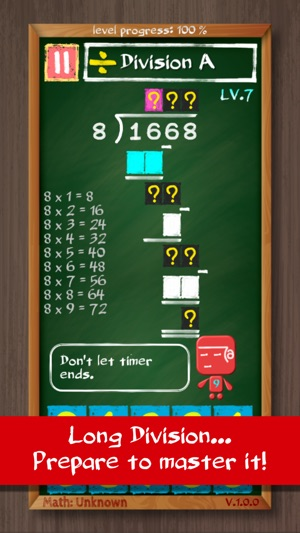## Description

If you're a student.. Are you struggling with the following math problems?
+ Addition: Forgot carrying (regrouping)
- Subtraction: Couldn't visualize how borrowing works
× Multiplication: Don't know where to put what
÷ Division: Don't know how to do a long division

If you're a math teacher or parents...
Would you like to have a tool that can help you teach and guide your kids?

Let's find out how this math game will help you!

"Math: Unknown" is a step-by-step math calculation game which will teach players "how" to calculate in the correct order.

Unknowns are numbers you have to solve.
The more you solve unknowns, the more you know.

You will focus on 4 kinds of math operations i.e. addition, subtraction, multiplication and long division. It's like having your personal math tutor with you anywhere.. anytime.

The game has 4 main arithmetic operations. Each operation has 2 sets (A and B) and each set contains 8 levels of difficulty with unlimited number of problems. So you can play as many as you want, there is no limitation. The score (stars) you got will be accumulated for each set to compete with other players via Game Center.

=== FEATURES ===
+ Step-by-step calculations as doing it on paper -- NOT just asking for final result
+ Calculation hints for some levels
+ Wrong answer will always be corrected
+ Calculation guides for quick reference
+ Multiplication table
+ 8 Operation sets
+ 8 Levels for each set
+ Unlimited number of questions
+ Basic calculation on set A
+ More challenging calculation on set B
+ Progress Summary of each 4 main operations
+ Number of wins vs total plays as well as winning percentage of each level
+ Game Center -- Leaderboards and Achievement
+ Speed bonus for fast calculation head
+ No time constrains. Take your time before answer.
+ Level completion bonus
+ Feel-at-school theme: blackboard, chalk and hand-drawn font
+ "Mu" will be your partner to cheer you up

=== HIGHLIGHTS ===
[+] Addition with carrying (regrouping)
[-] Subtraction with borrowing
[×] Detailed Multiplication
[÷] Long Division

=== DETAILS ===
For all questions, if you did it wrong, there will be corrections to show the correct answers

+ Starting from basic addition
+ Gradually increases difficulty -- more number of digits
+ Automatically show carrying (regrouping) when necessary just to let you see how it is done on the paper.
+ Once you're familiar with carrying, you'll have to do it all yourself in higher levels.

 Subtraction:
- Starting with basic subtraction without borrowing
- Borrowing is needed in higher levels
- Borrowing is visualized by a single button press at the right time
- Visualized 2 ways of borrowing from the next place
-- Next place is a number with value more than 0
-- Next place is zero
- You'll learn when and how to borrow since the button will be active only at the right time
- Borrowing won't be shown in the later levels when you're familiar with it in order to let you do it all yourself

 Multiplication:
× Starting with basic
× More number of digits as level progresses
× Show carrying in early levels
× You don't have to do it all yourself, only a single row is needed to answer
× Relevant multiplication table is shown when necessary

 Division:
÷ Detailed Long Division
÷ Relevant multiplication table is shown so that you can quickly refer to it in division
÷ If you pick a correct quotient, the product (multiplication between divisor and a digit of quotient) will be shown automatically. What you have to do is see the table and pick the right one
÷ You will have to subtract and pull a digit from dividend yourself
÷ You will have to calculate from top to the remainder
÷ There won't be any multiplication table in higher levels so that you can practice the real problem which you have to guess what will fit

 Calculation Guides:
+ Animated steps of solving all types of calculations
+ Multiplication table of number 2 to 19

Get it NOW and Start leveling up your math skills !

## Information

Seller
Suparerg Suksai
Size
44.1 MB
Category
Games
Compatibility

Requires iOS 8.0 or later. Compatible with iPhone, iPad, and iPod touch.

Languages

English

Age Rating
Rated 4+
© 2017 Suparerg Suksai
Price
\$4.99

## Supports

•### Game Center

#### Challenge friends and check leaderboards and achievements.

•